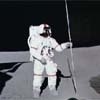#### You may also like### Lunar Leaper

Gravity on the Moon is about 1/6th that on the Earth. A pole-vaulter 2 metres tall can clear a 5 metres pole on the Earth. How high a pole could he clear on the Moon?### Which Twin Is Older?

A simplified account of special relativity and the twins paradox.### Whoosh

A ball whooshes down a slide and hits another ball which flies off the slide horizontally as a projectile. How far does it go?

# Ideal Gases

### Why do this problem?

This problem will get students thinking about the kinetic assumptions underlying ideal gasses. The problem draws together many different types of calculation, many involving non-standard estimations. Whilst there are many calculations to perform, the key purpose is to provoke thinking which will lead to an increased intuition of the natural ideas underlying ideal gasses.

### Possible approach

Some of the estimations are very difficult to perform with a high degree of certainty, although it is possible to put reasonable bounds on many of the quantities. The key point is the any assumptions made in any estimation are clearly made. For example, 'Assuming that the molecule travels with the average velocity ...' or 'Assuming that the molecule is moving perpendicularly to one of the faces ...' are good assumptions for starting some calculation.

Some students might feel uncomfortable with the lack of precise, exact numerical answers to many of the parts or with the fact that the problem will need standard data and equations sourced from elsewhere. This is good, as it will help to develop the independent thinking needed to succees with science and mathematics at higher levels.

### Key questions

What does your intuition tell you?

What assumptions have you made in each case?